Anúncio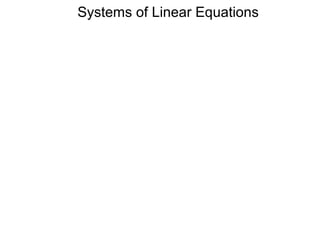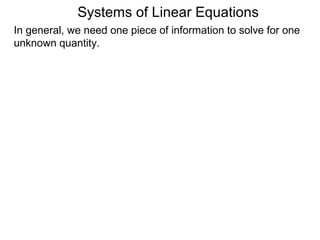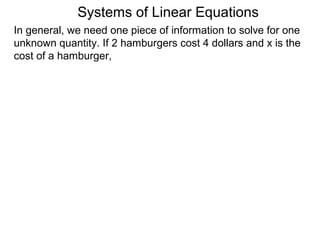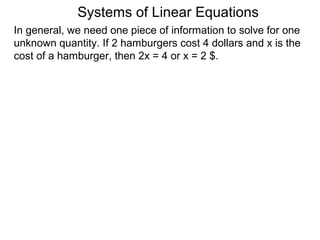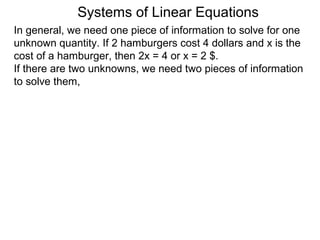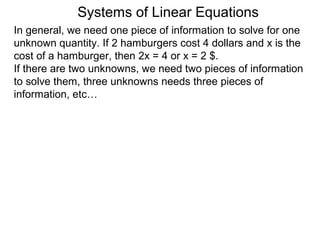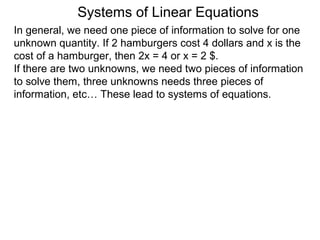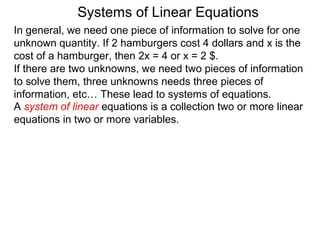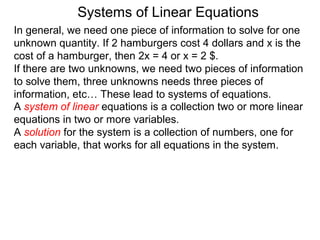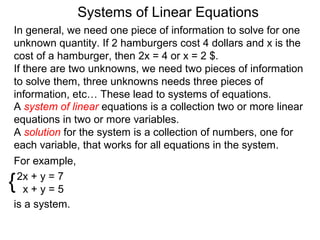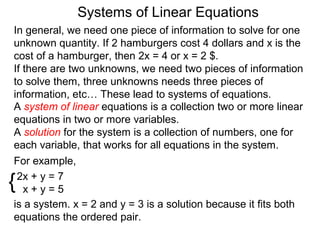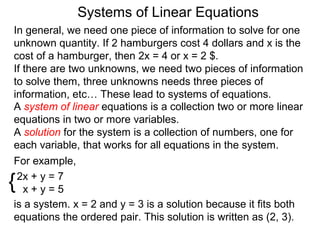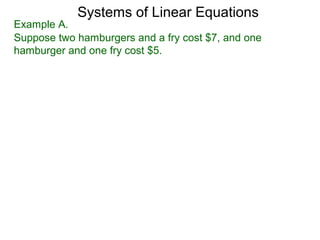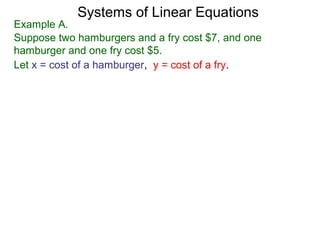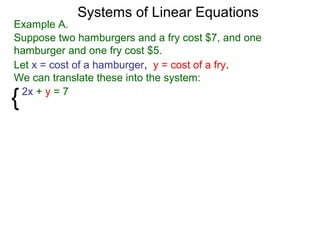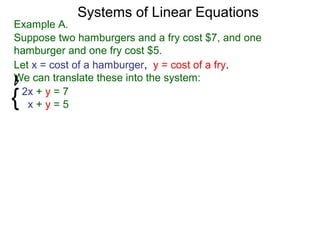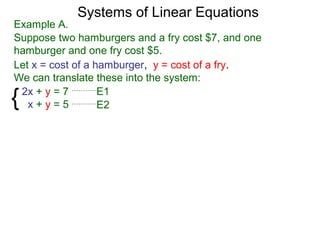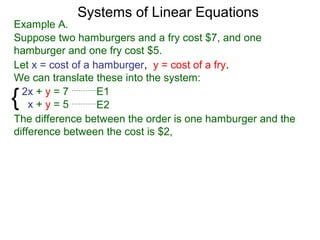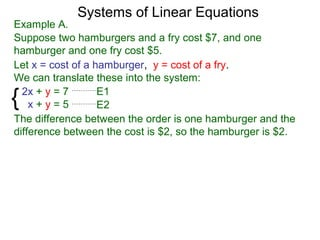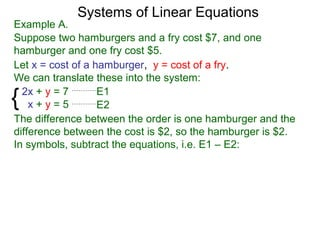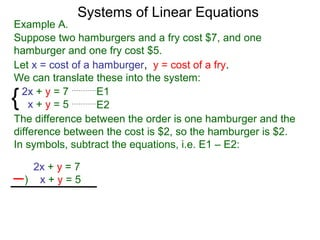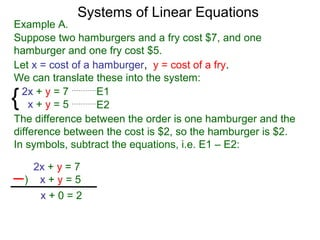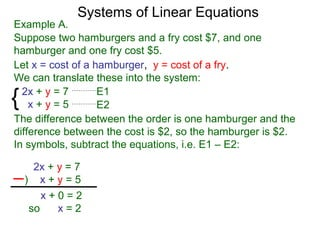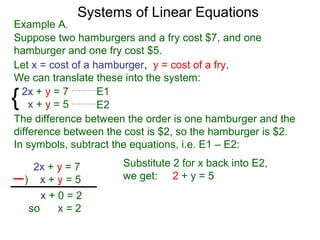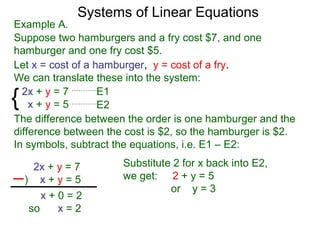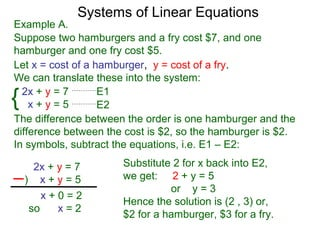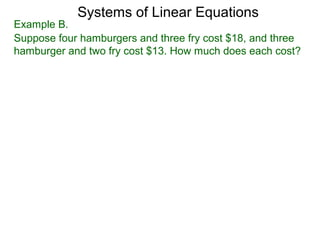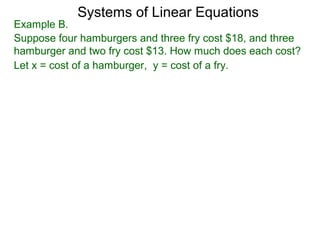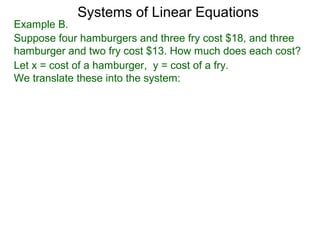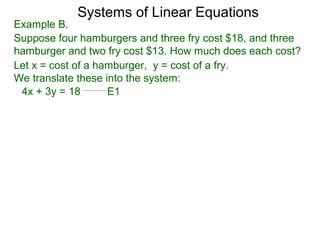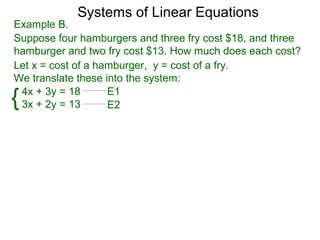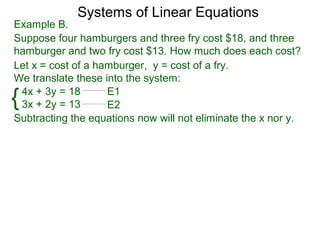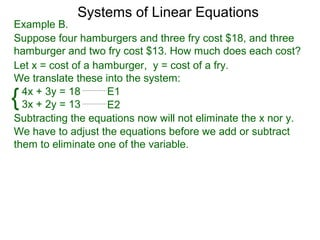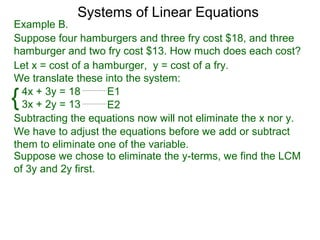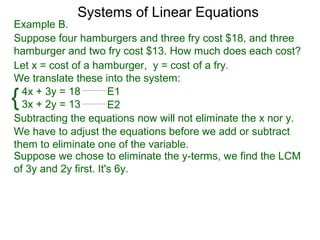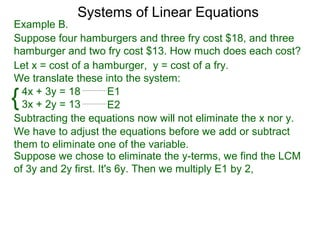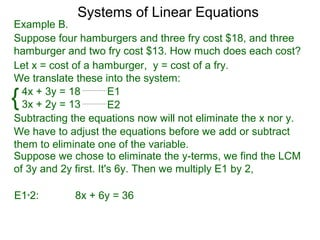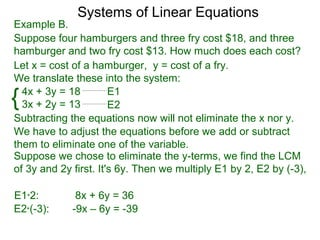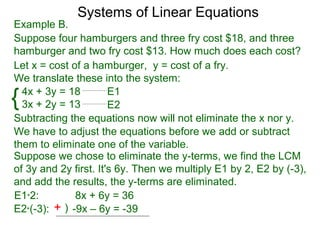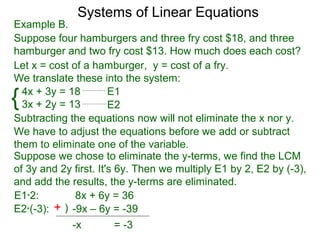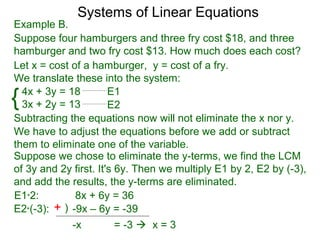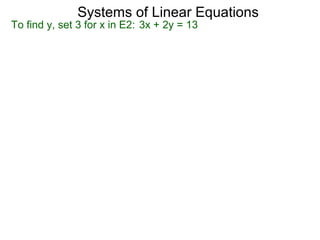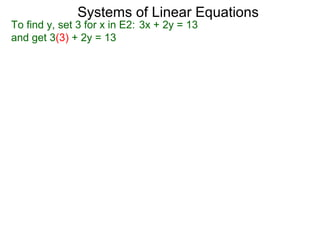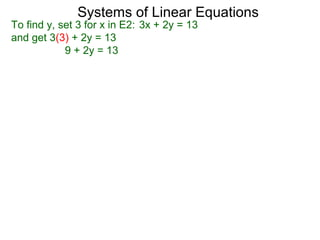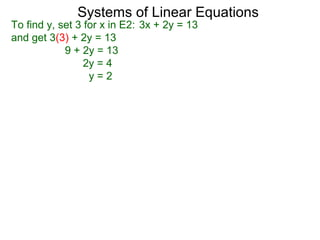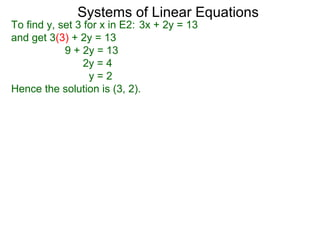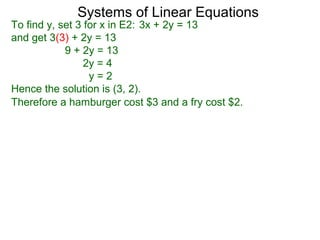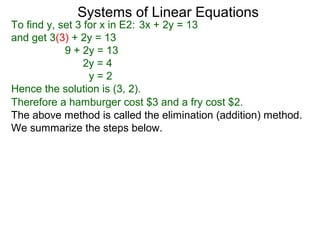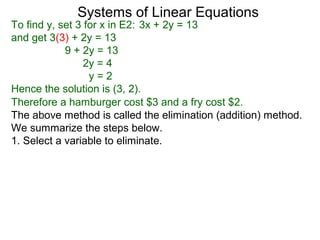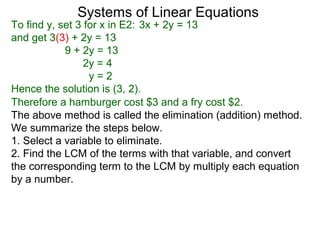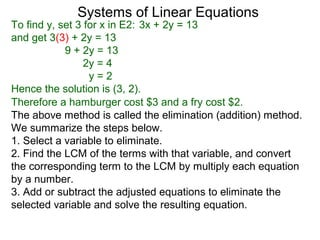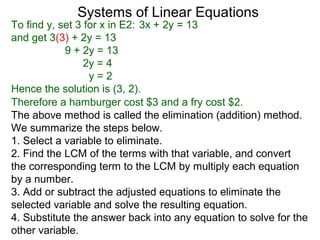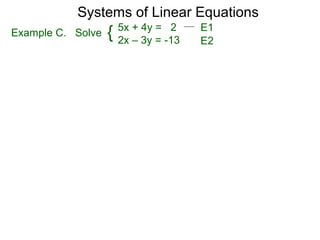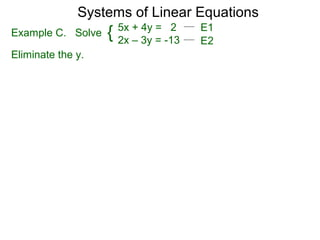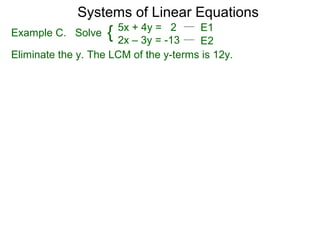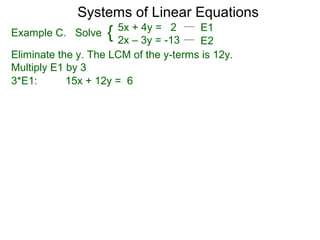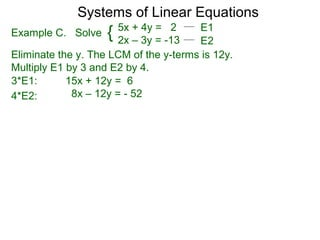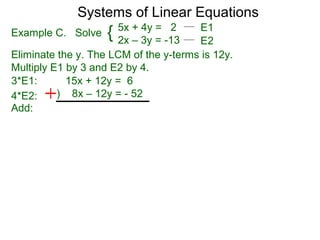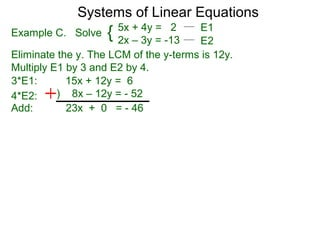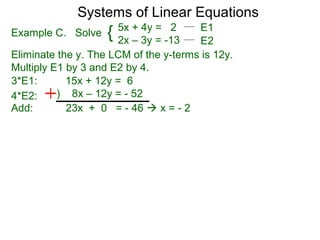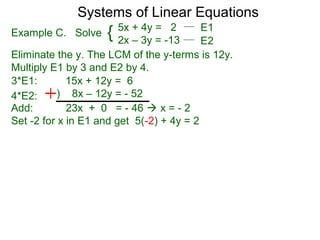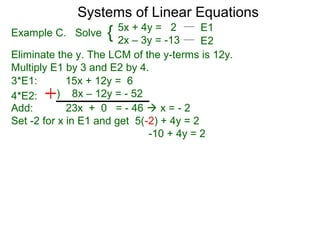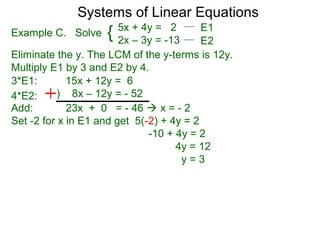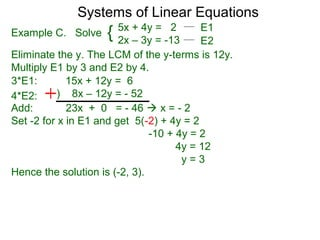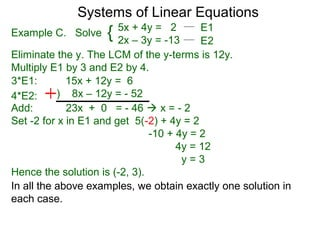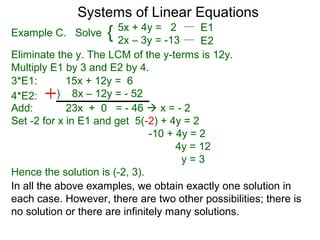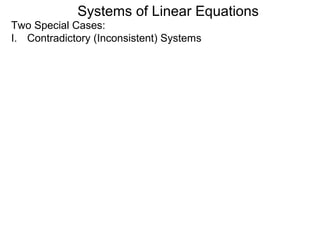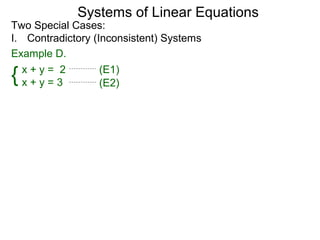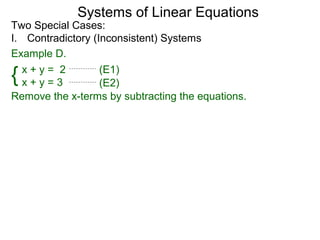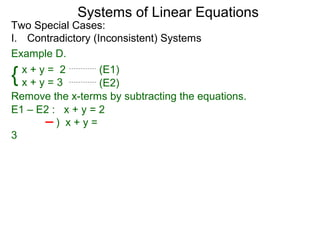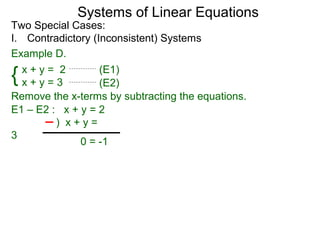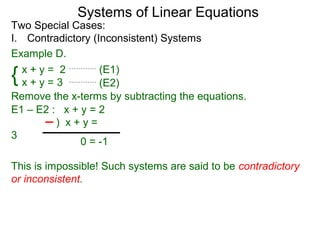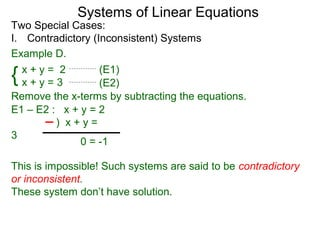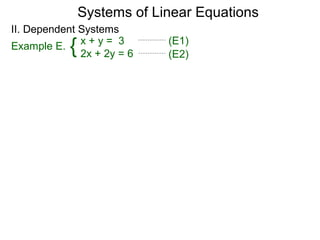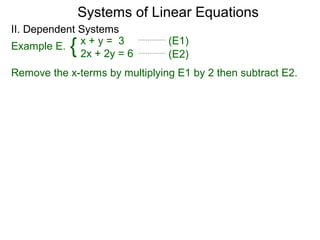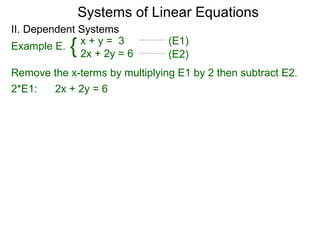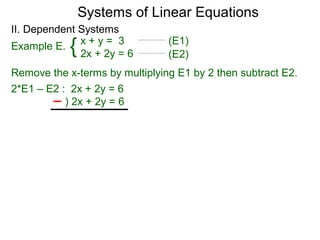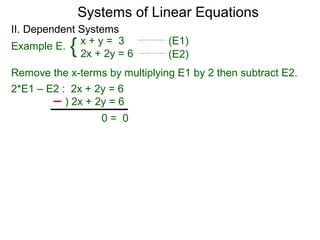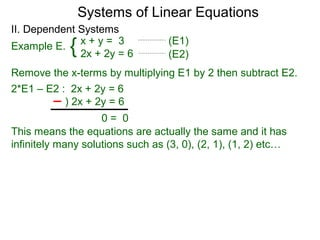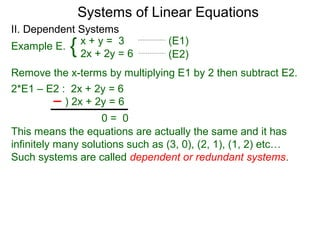1 de 80
Anúncio

### 81 systems of linear equations 1

1. Systems of Linear Equations
2. Systems of Linear Equations In general, we need one piece of information to solve for one unknown quantity.
3. Systems of Linear Equations In general, we need one piece of information to solve for one unknown quantity. If 2 hamburgers cost 4 dollars and x is the cost of a hamburger,
4. Systems of Linear Equations In general, we need one piece of information to solve for one unknown quantity. If 2 hamburgers cost 4 dollars and x is the cost of a hamburger, then 2x = 4 or x = 2 \$.
5. Systems of Linear Equations In general, we need one piece of information to solve for one unknown quantity. If 2 hamburgers cost 4 dollars and x is the cost of a hamburger, then 2x = 4 or x = 2 \$. If there are two unknowns, we need two pieces of information to solve them,
6. Systems of Linear Equations In general, we need one piece of information to solve for one unknown quantity. If 2 hamburgers cost 4 dollars and x is the cost of a hamburger, then 2x = 4 or x = 2 \$. If there are two unknowns, we need two pieces of information to solve them, three unknowns needs three pieces of information, etc…
7. Systems of Linear Equations In general, we need one piece of information to solve for one unknown quantity. If 2 hamburgers cost 4 dollars and x is the cost of a hamburger, then 2x = 4 or x = 2 \$. If there are two unknowns, we need two pieces of information to solve them, three unknowns needs three pieces of information, etc… These lead to systems of equations.
8. Systems of Linear Equations In general, we need one piece of information to solve for one unknown quantity. If 2 hamburgers cost 4 dollars and x is the cost of a hamburger, then 2x = 4 or x = 2 \$. If there are two unknowns, we need two pieces of information to solve them, three unknowns needs three pieces of information, etc… These lead to systems of equations. A system of linear equations is a collection two or more linear equations in two or more variables.
9. Systems of Linear Equations In general, we need one piece of information to solve for one unknown quantity. If 2 hamburgers cost 4 dollars and x is the cost of a hamburger, then 2x = 4 or x = 2 \$. If there are two unknowns, we need two pieces of information to solve them, three unknowns needs three pieces of information, etc… These lead to systems of equations. A system of linear equations is a collection two or more linear equations in two or more variables. A solution for the system is a collection of numbers, one for each variable, that works for all equations in the system.
10. Systems of Linear Equations In general, we need one piece of information to solve for one unknown quantity. If 2 hamburgers cost 4 dollars and x is the cost of a hamburger, then 2x = 4 or x = 2 \$. If there are two unknowns, we need two pieces of information to solve them, three unknowns needs three pieces of information, etc… These lead to systems of equations. A system of linear equations is a collection two or more linear equations in two or more variables. A solution for the system is a collection of numbers, one for each variable, that works for all equations in the system. For example, {2x + y = 7 x+y=5 is a system.
11. Systems of Linear Equations In general, we need one piece of information to solve for one unknown quantity. If 2 hamburgers cost 4 dollars and x is the cost of a hamburger, then 2x = 4 or x = 2 \$. If there are two unknowns, we need two pieces of information to solve them, three unknowns needs three pieces of information, etc… These lead to systems of equations. A system of linear equations is a collection two or more linear equations in two or more variables. A solution for the system is a collection of numbers, one for each variable, that works for all equations in the system. For example, {2x + y = 7 x+y=5 is a system. x = 2 and y = 3 is a solution because it fits both equations the ordered pair.
12. Systems of Linear Equations In general, we need one piece of information to solve for one unknown quantity. If 2 hamburgers cost 4 dollars and x is the cost of a hamburger, then 2x = 4 or x = 2 \$. If there are two unknowns, we need two pieces of information to solve them, three unknowns needs three pieces of information, etc… These lead to systems of equations. A system of linear equations is a collection two or more linear equations in two or more variables. A solution for the system is a collection of numbers, one for each variable, that works for all equations in the system. For example, {2x + y = 7 x+y=5 is a system. x = 2 and y = 3 is a solution because it fits both equations the ordered pair. This solution is written as (2, 3).
13. Systems of Linear Equations Example A. Suppose two hamburgers and a fry cost \$7, and one hamburger and one fry cost \$5.
14. Systems of Linear Equations Example A. Suppose two hamburgers and a fry cost \$7, and one hamburger and one fry cost \$5. Let x = cost of a hamburger, y = cost of a fry.
15. Systems of Linear Equations Example A. Suppose two hamburgers and a fry cost \$7, and one hamburger and one fry cost \$5. Let x = cost of a hamburger, y = cost of a fry. We can translate these into the system: {2x + y = 7
16. Systems of Linear Equations Example A. Suppose two hamburgers and a fry cost \$7, and one hamburger and one fry cost \$5. Let x = cost of a hamburger, y = cost of a fry. We can translate these into the system: {2x + y = 7 x+y=5
17. Systems of Linear Equations Example A. Suppose two hamburgers and a fry cost \$7, and one hamburger and one fry cost \$5. Let x = cost of a hamburger, y = cost of a fry. We can translate these into the system: {2x + y = 7 x+y=5 E1 E2
18. Systems of Linear Equations Example A. Suppose two hamburgers and a fry cost \$7, and one hamburger and one fry cost \$5. Let x = cost of a hamburger, y = cost of a fry. We can translate these into the system: { 2x + y = 7 x+y=5 E1 E2 The difference between the order is one hamburger and the difference between the cost is \$2,
19. Systems of Linear Equations Example A. Suppose two hamburgers and a fry cost \$7, and one hamburger and one fry cost \$5. Let x = cost of a hamburger, y = cost of a fry. We can translate these into the system: { 2x + y = 7 x+y=5 E1 E2 The difference between the order is one hamburger and the difference between the cost is \$2, so the hamburger is \$2.
20. Systems of Linear Equations Example A. Suppose two hamburgers and a fry cost \$7, and one hamburger and one fry cost \$5. Let x = cost of a hamburger, y = cost of a fry. We can translate these into the system: { 2x + y = 7 x+y=5 E1 E2 The difference between the order is one hamburger and the difference between the cost is \$2, so the hamburger is \$2. In symbols, subtract the equations, i.e. E1 – E2:
21. Systems of Linear Equations Example A. Suppose two hamburgers and a fry cost \$7, and one hamburger and one fry cost \$5. Let x = cost of a hamburger, y = cost of a fry. We can translate these into the system: { 2x + y = 7 x+y=5 E1 E2 The difference between the order is one hamburger and the difference between the cost is \$2, so the hamburger is \$2. In symbols, subtract the equations, i.e. E1 – E2: 2x + y = 7 ) x+y=5
22. Systems of Linear Equations Example A. Suppose two hamburgers and a fry cost \$7, and one hamburger and one fry cost \$5. Let x = cost of a hamburger, y = cost of a fry. We can translate these into the system: { 2x + y = 7 x+y=5 E1 E2 The difference between the order is one hamburger and the difference between the cost is \$2, so the hamburger is \$2. In symbols, subtract the equations, i.e. E1 – E2: 2x + y = 7 ) x+y=5 x+0=2
23. Systems of Linear Equations Example A. Suppose two hamburgers and a fry cost \$7, and one hamburger and one fry cost \$5. Let x = cost of a hamburger, y = cost of a fry. We can translate these into the system: { 2x + y = 7 x+y=5 E1 E2 The difference between the order is one hamburger and the difference between the cost is \$2, so the hamburger is \$2. In symbols, subtract the equations, i.e. E1 – E2: 2x + y = 7 ) x+y=5 x+0=2 so x=2
24. Systems of Linear Equations Example A. Suppose two hamburgers and a fry cost \$7, and one hamburger and one fry cost \$5. Let x = cost of a hamburger, y = cost of a fry. We can translate these into the system: { 2x + y = 7 x+y=5 E1 E2 The difference between the order is one hamburger and the difference between the cost is \$2, so the hamburger is \$2. In symbols, subtract the equations, i.e. E1 – E2: 2x + y = 7 Substitute 2 for x back into E2, ) x+y=5 we get: 2 + y = 5 x+0=2 so x=2
25. Systems of Linear Equations Example A. Suppose two hamburgers and a fry cost \$7, and one hamburger and one fry cost \$5. Let x = cost of a hamburger, y = cost of a fry. We can translate these into the system: { 2x + y = 7 x+y=5 E1 E2 The difference between the order is one hamburger and the difference between the cost is \$2, so the hamburger is \$2. In symbols, subtract the equations, i.e. E1 – E2: 2x + y = 7 Substitute 2 for x back into E2, ) x+y=5 we get: 2 + y = 5 or y = 3 x+0=2 so x=2
26. Systems of Linear Equations Example A. Suppose two hamburgers and a fry cost \$7, and one hamburger and one fry cost \$5. Let x = cost of a hamburger, y = cost of a fry. We can translate these into the system: { 2x + y = 7 x+y=5 E1 E2 The difference between the order is one hamburger and the difference between the cost is \$2, so the hamburger is \$2. In symbols, subtract the equations, i.e. E1 – E2: 2x + y = 7 Substitute 2 for x back into E2, ) x+y=5 we get: 2 + y = 5 or y = 3 x+0=2 Hence the solution is (2 , 3) or, so x=2 \$2 for a hamburger, \$3 for a fry.
27. Systems of Linear Equations Example B. Suppose four hamburgers and three fry cost \$18, and three hamburger and two fry cost \$13. How much does each cost?
28. Systems of Linear Equations Example B. Suppose four hamburgers and three fry cost \$18, and three hamburger and two fry cost \$13. How much does each cost? Let x = cost of a hamburger, y = cost of a fry.
29. Systems of Linear Equations Example B. Suppose four hamburgers and three fry cost \$18, and three hamburger and two fry cost \$13. How much does each cost? Let x = cost of a hamburger, y = cost of a fry. We translate these into the system:
30. Systems of Linear Equations Example B. Suppose four hamburgers and three fry cost \$18, and three hamburger and two fry cost \$13. How much does each cost? Let x = cost of a hamburger, y = cost of a fry. We translate these into the system: 4x + 3y = 18 E1
31. Systems of Linear Equations Example B. Suppose four hamburgers and three fry cost \$18, and three hamburger and two fry cost \$13. How much does each cost? Let x = cost of a hamburger, y = cost of a fry. We translate these into the system: {4x + 3y = 18 3x + 2y = 13 E1 E2
32. Systems of Linear Equations Example B. Suppose four hamburgers and three fry cost \$18, and three hamburger and two fry cost \$13. How much does each cost? Let x = cost of a hamburger, y = cost of a fry. We translate these into the system: {4x + 3y = 18 3x + 2y = 13 E1 E2 Subtracting the equations now will not eliminate the x nor y.
33. Systems of Linear Equations Example B. Suppose four hamburgers and three fry cost \$18, and three hamburger and two fry cost \$13. How much does each cost? Let x = cost of a hamburger, y = cost of a fry. We translate these into the system: { 4x + 3y = 18 3x + 2y = 13 E1 E2 Subtracting the equations now will not eliminate the x nor y. We have to adjust the equations before we add or subtract them to eliminate one of the variable.
34. Systems of Linear Equations Example B. Suppose four hamburgers and three fry cost \$18, and three hamburger and two fry cost \$13. How much does each cost? Let x = cost of a hamburger, y = cost of a fry. We translate these into the system: { 4x + 3y = 18 3x + 2y = 13 E1 E2 Subtracting the equations now will not eliminate the x nor y. We have to adjust the equations before we add or subtract them to eliminate one of the variable. Suppose we chose to eliminate the y-terms, we find the LCM of 3y and 2y first.
35. Systems of Linear Equations Example B. Suppose four hamburgers and three fry cost \$18, and three hamburger and two fry cost \$13. How much does each cost? Let x = cost of a hamburger, y = cost of a fry. We translate these into the system: { 4x + 3y = 18 3x + 2y = 13 E1 E2 Subtracting the equations now will not eliminate the x nor y. We have to adjust the equations before we add or subtract them to eliminate one of the variable. Suppose we chose to eliminate the y-terms, we find the LCM of 3y and 2y first. It's 6y.
36. Systems of Linear Equations Example B. Suppose four hamburgers and three fry cost \$18, and three hamburger and two fry cost \$13. How much does each cost? Let x = cost of a hamburger, y = cost of a fry. We translate these into the system: { 4x + 3y = 18 3x + 2y = 13 E1 E2 Subtracting the equations now will not eliminate the x nor y. We have to adjust the equations before we add or subtract them to eliminate one of the variable. Suppose we chose to eliminate the y-terms, we find the LCM of 3y and 2y first. It's 6y. Then we multiply E1 by 2,
37. Systems of Linear Equations Example B. Suppose four hamburgers and three fry cost \$18, and three hamburger and two fry cost \$13. How much does each cost? Let x = cost of a hamburger, y = cost of a fry. We translate these into the system: { 4x + 3y = 18 3x + 2y = 13 E1 E2 Subtracting the equations now will not eliminate the x nor y. We have to adjust the equations before we add or subtract them to eliminate one of the variable. Suppose we chose to eliminate the y-terms, we find the LCM of 3y and 2y first. It's 6y. Then we multiply E1 by 2, E1*2: 8x + 6y = 36
38. Systems of Linear Equations Example B. Suppose four hamburgers and three fry cost \$18, and three hamburger and two fry cost \$13. How much does each cost? Let x = cost of a hamburger, y = cost of a fry. We translate these into the system: { 4x + 3y = 18 3x + 2y = 13 E1 E2 Subtracting the equations now will not eliminate the x nor y. We have to adjust the equations before we add or subtract them to eliminate one of the variable. Suppose we chose to eliminate the y-terms, we find the LCM of 3y and 2y first. It's 6y. Then we multiply E1 by 2, E2 by (-3), E1*2: 8x + 6y = 36 E2*(-3): -9x – 6y = -39
39. Systems of Linear Equations Example B. Suppose four hamburgers and three fry cost \$18, and three hamburger and two fry cost \$13. How much does each cost? Let x = cost of a hamburger, y = cost of a fry. We translate these into the system: { 4x + 3y = 18 3x + 2y = 13 E1 E2 Subtracting the equations now will not eliminate the x nor y. We have to adjust the equations before we add or subtract them to eliminate one of the variable. Suppose we chose to eliminate the y-terms, we find the LCM of 3y and 2y first. It's 6y. Then we multiply E1 by 2, E2 by (-3), and add the results, the y-terms are eliminated. E1*2: 8x + 6y = 36 E2*(-3): + ) -9x – 6y = -39
40. Systems of Linear Equations Example B. Suppose four hamburgers and three fry cost \$18, and three hamburger and two fry cost \$13. How much does each cost? Let x = cost of a hamburger, y = cost of a fry. We translate these into the system: { 4x + 3y = 18 3x + 2y = 13 E1 E2 Subtracting the equations now will not eliminate the x nor y. We have to adjust the equations before we add or subtract them to eliminate one of the variable. Suppose we chose to eliminate the y-terms, we find the LCM of 3y and 2y first. It's 6y. Then we multiply E1 by 2, E2 by (-3), and add the results, the y-terms are eliminated. E1*2: 8x + 6y = 36 E2*(-3): + ) -9x – 6y = -39 -x = -3
41. Systems of Linear Equations Example B. Suppose four hamburgers and three fry cost \$18, and three hamburger and two fry cost \$13. How much does each cost? Let x = cost of a hamburger, y = cost of a fry. We translate these into the system: { 4x + 3y = 18 3x + 2y = 13 E1 E2 Subtracting the equations now will not eliminate the x nor y. We have to adjust the equations before we add or subtract them to eliminate one of the variable. Suppose we chose to eliminate the y-terms, we find the LCM of 3y and 2y first. It's 6y. Then we multiply E1 by 2, E2 by (-3), and add the results, the y-terms are eliminated. E1*2: 8x + 6y = 36 E2*(-3): + ) -9x – 6y = -39 -x = -3  x = 3
42. Systems of Linear Equations To find y, set 3 for x in E2: 3x + 2y = 13
43. Systems of Linear Equations To find y, set 3 for x in E2: 3x + 2y = 13 and get 3(3) + 2y = 13
44. Systems of Linear Equations To find y, set 3 for x in E2: 3x + 2y = 13 and get 3(3) + 2y = 13 9 + 2y = 13
45. Systems of Linear Equations To find y, set 3 for x in E2: 3x + 2y = 13 and get 3(3) + 2y = 13 9 + 2y = 13 2y = 4 y=2
46. Systems of Linear Equations To find y, set 3 for x in E2: 3x + 2y = 13 and get 3(3) + 2y = 13 9 + 2y = 13 2y = 4 y=2 Hence the solution is (3, 2).
47. Systems of Linear Equations To find y, set 3 for x in E2: 3x + 2y = 13 and get 3(3) + 2y = 13 9 + 2y = 13 2y = 4 y=2 Hence the solution is (3, 2). Therefore a hamburger cost \$3 and a fry cost \$2.
48. Systems of Linear Equations To find y, set 3 for x in E2: 3x + 2y = 13 and get 3(3) + 2y = 13 9 + 2y = 13 2y = 4 y=2 Hence the solution is (3, 2). Therefore a hamburger cost \$3 and a fry cost \$2. The above method is called the elimination (addition) method. We summarize the steps below.
49. Systems of Linear Equations To find y, set 3 for x in E2: 3x + 2y = 13 and get 3(3) + 2y = 13 9 + 2y = 13 2y = 4 y=2 Hence the solution is (3, 2). Therefore a hamburger cost \$3 and a fry cost \$2. The above method is called the elimination (addition) method. We summarize the steps below. 1. Select a variable to eliminate.
50. Systems of Linear Equations To find y, set 3 for x in E2: 3x + 2y = 13 and get 3(3) + 2y = 13 9 + 2y = 13 2y = 4 y=2 Hence the solution is (3, 2). Therefore a hamburger cost \$3 and a fry cost \$2. The above method is called the elimination (addition) method. We summarize the steps below. 1. Select a variable to eliminate. 2. Find the LCM of the terms with that variable, and convert the corresponding term to the LCM by multiply each equation by a number.
51. Systems of Linear Equations To find y, set 3 for x in E2: 3x + 2y = 13 and get 3(3) + 2y = 13 9 + 2y = 13 2y = 4 y=2 Hence the solution is (3, 2). Therefore a hamburger cost \$3 and a fry cost \$2. The above method is called the elimination (addition) method. We summarize the steps below. 1. Select a variable to eliminate. 2. Find the LCM of the terms with that variable, and convert the corresponding term to the LCM by multiply each equation by a number. 3. Add or subtract the adjusted equations to eliminate the selected variable and solve the resulting equation.
52. Systems of Linear Equations To find y, set 3 for x in E2: 3x + 2y = 13 and get 3(3) + 2y = 13 9 + 2y = 13 2y = 4 y=2 Hence the solution is (3, 2). Therefore a hamburger cost \$3 and a fry cost \$2. The above method is called the elimination (addition) method. We summarize the steps below. 1. Select a variable to eliminate. 2. Find the LCM of the terms with that variable, and convert the corresponding term to the LCM by multiply each equation by a number. 3. Add or subtract the adjusted equations to eliminate the selected variable and solve the resulting equation. 4. Substitute the answer back into any equation to solve for the other variable.
53. Systems of Linear Equations 5x + 4y = 2 E1 Example C. Solve { 2x – 3y = -13 E2
54. Systems of Linear Equations 5x + 4y = 2 E1 Example C. Solve { 2x – 3y = -13 E2 Eliminate the y.
55. Systems of Linear Equations 5x + 4y = 2 E1 Example C. Solve { 2x – 3y = -13 E2 Eliminate the y. The LCM of the y-terms is 12y.
56. Systems of Linear Equations 5x + 4y = 2 E1 Example C. Solve { 2x – 3y = -13 E2 Eliminate the y. The LCM of the y-terms is 12y. Multiply E1 by 3 3*E1: 15x + 12y = 6
57. Systems of Linear Equations 5x + 4y = 2 E1 Example C. Solve { 2x – 3y = -13 E2 Eliminate the y. The LCM of the y-terms is 12y. Multiply E1 by 3 and E2 by 4. 3*E1: 15x + 12y = 6 4*E2: 8x – 12y = - 52
58. Systems of Linear Equations 5x + 4y = 2 E1 Example C. Solve { 2x – 3y = -13 E2 Eliminate the y. The LCM of the y-terms is 12y. Multiply E1 by 3 and E2 by 4. 3*E1: 15x + 12y = 6 4*E2: ) 8x – 12y = - 52 Add:
59. Systems of Linear Equations 5x + 4y = 2 E1 Example C. Solve { 2x – 3y = -13 E2 Eliminate the y. The LCM of the y-terms is 12y. Multiply E1 by 3 and E2 by 4. 3*E1: 15x + 12y = 6 4*E2: ) 8x – 12y = - 52 Add: 23x + 0 = - 46
60. Systems of Linear Equations 5x + 4y = 2 E1 Example C. Solve { 2x – 3y = -13 E2 Eliminate the y. The LCM of the y-terms is 12y. Multiply E1 by 3 and E2 by 4. 3*E1: 15x + 12y = 6 4*E2: ) 8x – 12y = - 52 Add: 23x + 0 = - 46  x = - 2
61. Systems of Linear Equations 5x + 4y = 2 E1 Example C. Solve { 2x – 3y = -13 E2 Eliminate the y. The LCM of the y-terms is 12y. Multiply E1 by 3 and E2 by 4. 3*E1: 15x + 12y = 6 4*E2: ) 8x – 12y = - 52 Add: 23x + 0 = - 46  x = - 2 Set -2 for x in E1 and get 5(-2) + 4y = 2
62. Systems of Linear Equations 5x + 4y = 2 E1 Example C. Solve { 2x – 3y = -13 E2 Eliminate the y. The LCM of the y-terms is 12y. Multiply E1 by 3 and E2 by 4. 3*E1: 15x + 12y = 6 4*E2: ) 8x – 12y = - 52 Add: 23x + 0 = - 46  x = - 2 Set -2 for x in E1 and get 5(-2) + 4y = 2 -10 + 4y = 2
63. Systems of Linear Equations 5x + 4y = 2 E1 Example C. Solve { 2x – 3y = -13 E2 Eliminate the y. The LCM of the y-terms is 12y. Multiply E1 by 3 and E2 by 4. 3*E1: 15x + 12y = 6 4*E2: ) 8x – 12y = - 52 Add: 23x + 0 = - 46  x = - 2 Set -2 for x in E1 and get 5(-2) + 4y = 2 -10 + 4y = 2 4y = 12 y=3
64. Systems of Linear Equations 5x + 4y = 2 E1 Example C. Solve { 2x – 3y = -13 E2 Eliminate the y. The LCM of the y-terms is 12y. Multiply E1 by 3 and E2 by 4. 3*E1: 15x + 12y = 6 4*E2: ) 8x – 12y = - 52 Add: 23x + 0 = - 46  x = - 2 Set -2 for x in E1 and get 5(-2) + 4y = 2 -10 + 4y = 2 4y = 12 y=3 Hence the solution is (-2, 3).
65. Systems of Linear Equations 5x + 4y = 2 E1 Example C. Solve { 2x – 3y = -13 E2 Eliminate the y. The LCM of the y-terms is 12y. Multiply E1 by 3 and E2 by 4. 3*E1: 15x + 12y = 6 4*E2: ) 8x – 12y = - 52 Add: 23x + 0 = - 46  x = - 2 Set -2 for x in E1 and get 5(-2) + 4y = 2 -10 + 4y = 2 4y = 12 y=3 Hence the solution is (-2, 3). In all the above examples, we obtain exactly one solution in each case.
66. Systems of Linear Equations 5x + 4y = 2 E1 Example C. Solve { 2x – 3y = -13 E2 Eliminate the y. The LCM of the y-terms is 12y. Multiply E1 by 3 and E2 by 4. 3*E1: 15x + 12y = 6 4*E2: ) 8x – 12y = - 52 Add: 23x + 0 = - 46  x = - 2 Set -2 for x in E1 and get 5(-2) + 4y = 2 -10 + 4y = 2 4y = 12 y=3 Hence the solution is (-2, 3). In all the above examples, we obtain exactly one solution in each case. However, there are two other possibilities; there is no solution or there are infinitely many solutions.
67. Systems of Linear Equations Two Special Cases: I. Contradictory (Inconsistent) Systems
68. Systems of Linear Equations Two Special Cases: I. Contradictory (Inconsistent) Systems Example D. { x+y= 2 x+y=3 (E1) (E2)
69. Systems of Linear Equations Two Special Cases: I. Contradictory (Inconsistent) Systems Example D. { x+y= 2 x+y=3 (E1) (E2) Remove the x-terms by subtracting the equations.
70. Systems of Linear Equations Two Special Cases: I. Contradictory (Inconsistent) Systems Example D. { x+y= 2 x+y=3 (E1) (E2) Remove the x-terms by subtracting the equations. E1 – E2 : x + y = 2 ) x+y= 3
71. Systems of Linear Equations Two Special Cases: I. Contradictory (Inconsistent) Systems Example D. { x+y= 2 x+y=3 (E1) (E2) Remove the x-terms by subtracting the equations. E1 – E2 : x + y = 2 ) x+y= 3 0 = -1
72. Systems of Linear Equations Two Special Cases: I. Contradictory (Inconsistent) Systems Example D. { x+y= 2 x+y=3 (E1) (E2) Remove the x-terms by subtracting the equations. E1 – E2 : x + y = 2 ) x+y= 3 0 = -1 This is impossible! Such systems are said to be contradictory or inconsistent.
73. Systems of Linear Equations Two Special Cases: I. Contradictory (Inconsistent) Systems Example D. { x+y= 2 x+y=3 (E1) (E2) Remove the x-terms by subtracting the equations. E1 – E2 : x + y = 2 ) x+y= 3 0 = -1 This is impossible! Such systems are said to be contradictory or inconsistent. These system don’t have solution.
74. Systems of Linear Equations II. Dependent Systems { Example E. x + y = 3 2x + 2y = 6 (E1) (E2)
75. Systems of Linear Equations II. Dependent Systems { Example E. x + y = 3 2x + 2y = 6 (E1) (E2) Remove the x-terms by multiplying E1 by 2 then subtract E2.
76. Systems of Linear Equations II. Dependent Systems { Example E. x + y = 3 2x + 2y = 6 (E1) (E2) Remove the x-terms by multiplying E1 by 2 then subtract E2. 2*E1: 2x + 2y = 6
77. Systems of Linear Equations II. Dependent Systems { Example E. x + y = 3 2x + 2y = 6 (E1) (E2) Remove the x-terms by multiplying E1 by 2 then subtract E2. 2*E1 – E2 : 2x + 2y = 6 ) 2x + 2y = 6
78. Systems of Linear Equations II. Dependent Systems { Example E. x + y = 3 2x + 2y = 6 (E1) (E2) Remove the x-terms by multiplying E1 by 2 then subtract E2. 2*E1 – E2 : 2x + 2y = 6 ) 2x + 2y = 6 0= 0
79. Systems of Linear Equations II. Dependent Systems { Example E. x + y = 3 2x + 2y = 6 (E1) (E2) Remove the x-terms by multiplying E1 by 2 then subtract E2. 2*E1 – E2 : 2x + 2y = 6 ) 2x + 2y = 6 0= 0 This means the equations are actually the same and it has infinitely many solutions such as (3, 0), (2, 1), (1, 2) etc…
80. Systems of Linear Equations II. Dependent Systems { Example E. x + y = 3 2x + 2y = 6 (E1) (E2) Remove the x-terms by multiplying E1 by 2 then subtract E2. 2*E1 – E2 : 2x + 2y = 6 ) 2x + 2y = 6 0= 0 This means the equations are actually the same and it has infinitely many solutions such as (3, 0), (2, 1), (1, 2) etc… Such systems are called dependent or redundant systems.
Anúncio